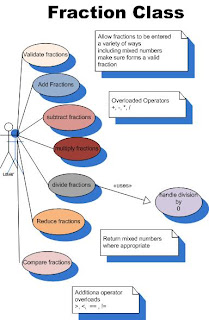## Thursday, October 8, 2009

### Fraction Class

So, last evening, with nothing on TV, I worked on developing a C# class called "Fraction" that will add, subtract, multiply, divide and compare fractions. This involves overloading several operators specifically (+,-,*,/,==,>,<,!=). The class has two integer fields, "numerator" and "denominator." Here is the Use Case diagram for the class:Here is the code for overloading the addition operator:

` public static Fraction operator +(Fraction F1, Fraction f2) {     if (F1.Denominator != f2.Denominator)     {         F1.Numerator = (F1.Numerator * f2.Denominator);         f2.Numerator = (f2.Numerator * F1.Denominator);         F1.Denominator = (F1.Denominator * f2.Denominator);         f2.Denominator = (F1.Denominator * f2.Denominator);       }     Fraction F3 = new Fraction();     F3.Numerator = F1.Numerator + f2.Numerator;     F3.Denominator = F1.Denominator;     return F3; }`

I would like to refactor the greatest common denominator code into a separate method since I also need to use it for subtraction, but I am not sure how to return the results I need to the operator method. I also intend to add a method to reduce the fraction, and a ToString() method that will output the fraction in a form like "1/2" o r"2 1/3."

This program isn't purely for my own amusement. It occurred to me some time ago, that this would be a great class to give to students to test. Each operation would need testing, and with a variety of inputs. Students could design a testing schema, conduct all the tests and document the results. It might make for a good special topics sometime.

On my book, I am almost finished with the Appendix on using Access with the book instead of SQL Express, though the hardest part is still to come. Security. Access security is nothing like SQL Server's security. I am tempted to just say, if you are using Access skip that chapter. But I will try to recreate the security measures in Access.

Now to water the lawn (a two or three hour task.)